## Hyperbolic Functions

The hyperbolic functions sinh, cosh, tanh, csch, sech, coth (Hyperbolic Sine, Hyperbolic Cosine, etc.) share many properties with the corresponding Circular Functions. The hyperbolic functions arise in many problems of mathematics and mathematical physics in which integrals involving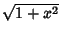arise (whereas the Circular Functions involve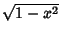).

For instance, the Hyperbolic Sine arises in the gravitational potential of a cylinderand the calculation of the Roche limit.The Hyperbolic Cosine function is the shape of a hanging cable (the so-called Catenary). The Hyperbolic Tangent arises in the calculation of magnetic momentand rapidityof special relativity.All three appear in the Schwarzschild metric using external isotropic Kruskal coordinatesin general relativity.The Hyperbolic Secant arises in the profile of a laminar jet.The Hyperbolic Cotangent arises in the Langevin functionfor magnetic polarization.The hyperbolic functions are defined by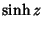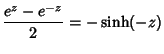(1)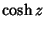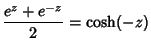(2)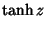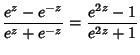(3)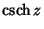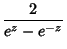(4)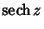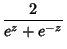(5)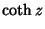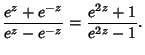(6)

For purely Imaginary arguments,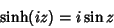(7)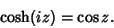(8)

The hyperbolic functions satisfy many identities anomalous to the trigonometric identities (which can be inferred using Osborne's Rule) such as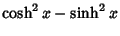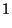(9)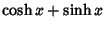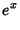(10)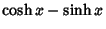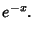(11)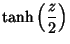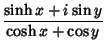(12)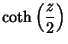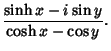(13)

Some double-angle Formulas are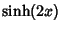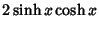(14)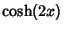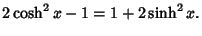(15)

Identities for Complex arguments include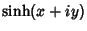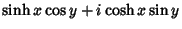(16)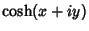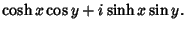(17)

The Absolute Squares for Complex arguments are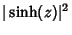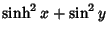(18)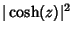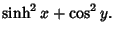(19)

Integrals involving hyperbolic functions include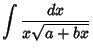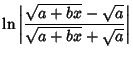(20)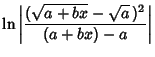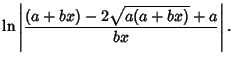(21)

If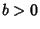, then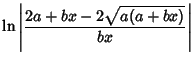(22)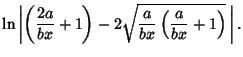(23)

Let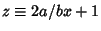, and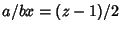and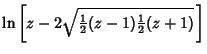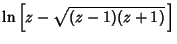(24)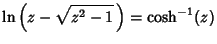(25)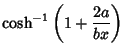(26)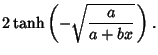(27)

See also Hyperbolic Cosecant, Hyperbolic Cosine, Hyperbolic Cotangent, Generalized Hyperbolic Functions, Hyperbolic Inverse Functions, Hyperbolic Secant, Hyperbolic Sine, Hyperbolic Tangent, Hyperbolic Inverse Functions, Osborne's Rule

References

Abramowitz, M. and Stegun, C. A. (Eds.). Hyperbolic Functions.'' §4.5 in Handbook of Mathematical Functions with Formulas, Graphs, and Mathematical Tables, 9th printing. New York: Dover, pp. 83-86, 1972.

Beyer, W. H. Hyperbolic Function.'' CRC Standard Mathematical Tables, 28th ed. Boca Raton, FL: CRC Press, pp. 168-186, 1987.

Coxeter, H. S. M. and Greitzer, S. L. Geometry Revisited. Washington, DC: Math. Assoc. Amer., pp. 126-131, 1967.

Yates, R. C. Hyperbolic Functions.'' A Handbook on Curves and Their Properties. Ann Arbor, MI: J. W. Edwards, pp. 113-118, 1952.